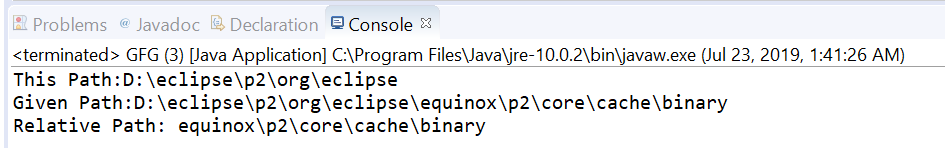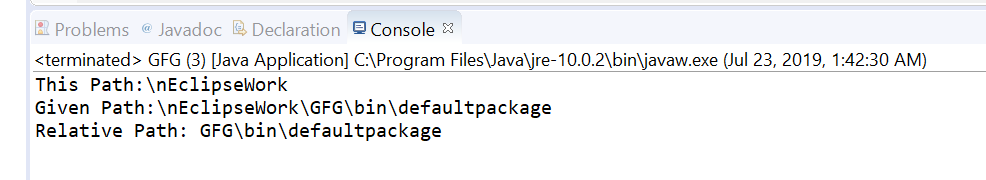# Path relativize() method in Java with Examples

The relativize(Path other) method of java.nio.file.Path used to create a relative path between this path and a given path as a parameter.
Relativization is the inverse of resolution. This method creates a relative path that when resolved against this path object, yields a path that helps us to locate the same file as the given path.

For example, if this path is “/dir1/dir2” and the given path as a parameter is “/dir1/dir2/dir3/file1” then this method will construct a relative path “dir3/file1”. Where this path and the given path do not have a root component, then a relative path can be constructed.

If anyone of the paths has a root component then the relative path cannot be constructed. When both paths have a root component then it is implementation-dependent if a relative path can be constructed. If this path and the given path are equal then an empty path is returned.

Syntax:

```Path relativize(Path other)
```

Parameters: This method accepts a one parameter other which is the the path to relativize against this path.

Return value: This method returns the resulting relative path, or an empty path if both paths are equal.

Exception: This method throws IllegalArgumentException if other is not a Path that can be relativized against this path.

Below programs illustrate relativize() method:
Program 1:

 `// Java program to demonstrate ` `// java.nio.file.Path.relativize() method ` ` `  `import` `java.nio.file.Path; ` `import` `java.nio.file.Paths; ` `public` `class` `GFG { ` `    ``public` `static` `void` `main(String[] args) ` `    ``{ ` ` `  `        ``// create objects of Path ` `        ``Path path ` `            ``= Paths.get(``"D:\\eclipse\\p2"` `                        ``+ ``"\\org\\eclipse"``); ` `        ``Path passedPath ` `            ``= Paths.get(``"D:\\eclipse\\p2"` `                        ``+ ``"\\org\\eclipse\\equinox\\p2\\core"` `                        ``+ ``"\\cache\\binary"``); ` ` `  `        ``// print paths ` `        ``System.out.println(``"This Path:"` `                           ``+ path); ` `        ``System.out.println(``"Given Path:"` `                           ``+ passedPath); ` ` `  `        ``// call relativize() to create ` `        ``// a relative path ` `        ``Path relativize ` `            ``= path.relativize(passedPath); ` ` `  `        ``// print result ` `        ``System.out.println(``"Relative Path: "` `                           ``+ relativize); ` `    ``} ` `} `

Output:Program 2:

 `// Java program to demonstrate ` `// java.nio.file.Path.relativize() method ` ` `  `import` `java.nio.file.Path; ` `import` `java.nio.file.Paths; ` `public` `class` `GFG { ` `    ``public` `static` `void` `main(String[] args) ` `    ``{ ` ` `  `        ``// create objects of Path ` `        ``Path path ` `            ``= Paths.get(``"\\nEclipseWork"``); ` `        ``Path passedPath ` `            ``= Paths.get(``"\\nEclipseWork\\GFG"` `                        ``+ ``"\\bin\\defaultpackage"``); ` ` `  `        ``// print paths ` `        ``System.out.println(``"This Path:"` `                           ``+ path); ` `        ``System.out.println(``"Given Path:"` `                           ``+ passedPath); ` ` `  `        ``// call relativize() ` `        ``// to create a relative path ` `        ``Path relativize ` `            ``= path.relativize(passedPath); ` ` `  `        ``// print result ` `        ``System.out.println(``"Relative Path: "` `                           ``+ relativize); ` `    ``} ` `} `

Output:Attention reader! Don’t stop learning now. Get hold of all the important Java and Collections concepts with the Fundamentals of Java and Java Collections Course at a student-friendly price and become industry ready.

My Personal Notes arrow_drop_upI am a Developer I love to code and bring my ideas alive

If you like GeeksforGeeks and would like to contribute, you can also write an article using contribute.geeksforgeeks.org or mail your article to contribute@geeksforgeeks.org. See your article appearing on the GeeksforGeeks main page and help other Geeks.

Please Improve this article if you find anything incorrect by clicking on the "Improve Article" button below.

Article Tags :
Practice Tags :

Be the First to upvote.

Please write to us at contribute@geeksforgeeks.org to report any issue with the above content.